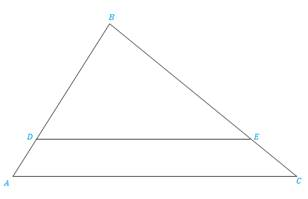Chapter 5.3, Problem 27E### Elementary Geometry for College St...

6th Edition
Daniel C. Alexander + 1 other
ISBN: 9781285195698

#### Solutions

Chapter
Section### Elementary Geometry for College St...

6th Edition
Daniel C. Alexander + 1 other
ISBN: 9781285195698
Textbook Problem
1 views

# In Exercises 25 to 28, Δ A B C ∼ Δ D B EExercises 25-28 Given: AC = 10, DE = 12, AD = 4. Find: DB

To determine

To find:

The side DB when the given sides are AC = 10, DE = 8, AD = 4 and ΔABCΔDBE.

Explanation

Definition:

CSSTP:

Corresponding sides of similar triangles are proportional.

Description:

The given figure is shown below.

Figure 1

From the given figure, it is observed that there are two triangles ABC and DBE and which is given that two triangles are similar.

Now draw the two triangles separately as shown below.

Figure 2

Figure 3

Since ΔABCΔDBE and from the definition of CSSTP, corresponding sides of similar triangles are proportional.

That is, BDBA=BEBC=DEAC.

It is given that AC = 10, DE = 8, AD = 4.

Find the side DB.

Consider the first and last two pairs of proportions DBAB=DEAC in order to find DB.

From Figure 1, it is observed that AB=AD+DB.

### Still sussing out bartleby?

Check out a sample textbook solution.

See a sample solution

#### The Solution to Your Study Problems

Bartleby provides explanations to thousands of textbook problems written by our experts, many with advanced degrees!

Get Started

#### In Exercises 19-24, find the functions f + g, f g, fg, and fg. 23. f(x) = x+1x1; g(x) = x+2x2

Applied Calculus for the Managerial, Life, and Social Sciences: A Brief Approach

#### Sum of Square (SS), variance and standard deviation

Statistics for The Behavioral Sciences (MindTap Course List)

#### Simplify: 5251155

Elementary Technical Mathematics

#### True or False: If and , then .

Study Guide for Stewart's Multivariable Calculus, 8th